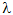# In a Young’s double slit experiment the intensity at a point where the path difference is /6(  being the wavelength of light used...

In a Young’s double slit experiment the intensity at a point where the path difference is/6(being the wavelength of light used) is I. If I0 denotes the maximum intensity, II0 is equal to

Anonymous User Physics Wave Optics 24 Apr, 2020 59 views